# Forces for extensions of meanfield Extensions of meanfield

• Slides: 16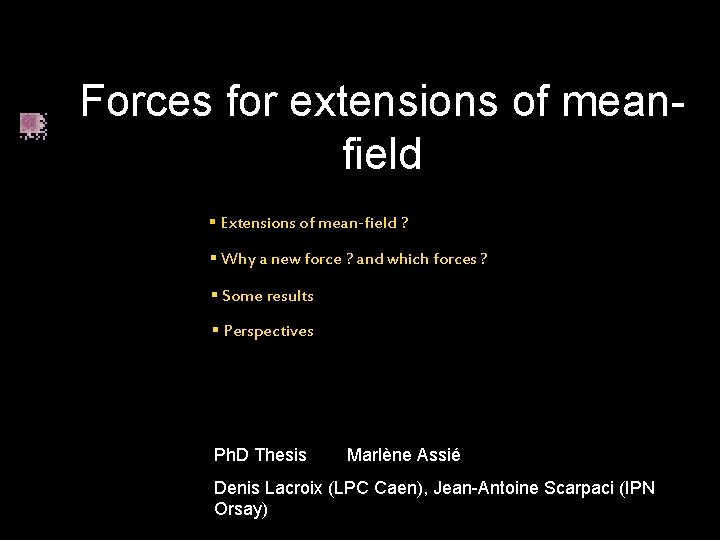Forces for extensions of meanfield § Extensions of mean-field ? § Why a new force ? and which forces ? § Some results § Perspectives Ph. D Thesis Marlène Assié Denis Lacroix (LPC Caen), Jean-Antoine Scarpaci (IPN Orsay)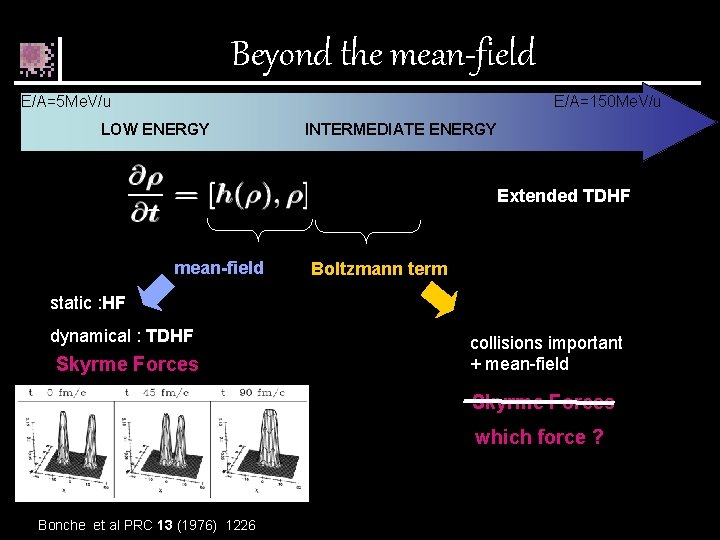Beyond the mean-field E/A=5 Me. V/u E/A=150 Me. V/u LOW ENERGY INTERMEDIATE ENERGY Extended TDHF mean-field Boltzmann term static : HF dynamical : TDHF Skyrme Forces collisions important + mean-field Skyrme Forces which force ? Bonche et al PRC 13 (1976) 1226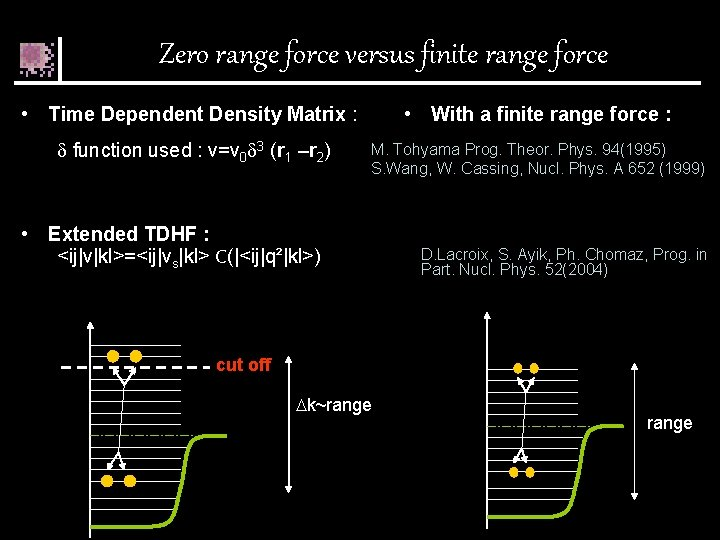Zero range force versus finite range force • Time Dependent Density Matrix : • With a finite range force : function used : v=v 0 3 (r 1 –r 2) M. Tohyama Prog. Theor. Phys. 94(1995) S. Wang, W. Cassing, Nucl. Phys. A 652 (1999) • Extended TDHF : <ij|v|kl>=<ij|vs|kl> C(|<ij|q²|kl>) D. Lacroix, S. Ayik, Ph. Chomaz, Prog. in Part. Nucl. Phys. 52(2004) cut off k~range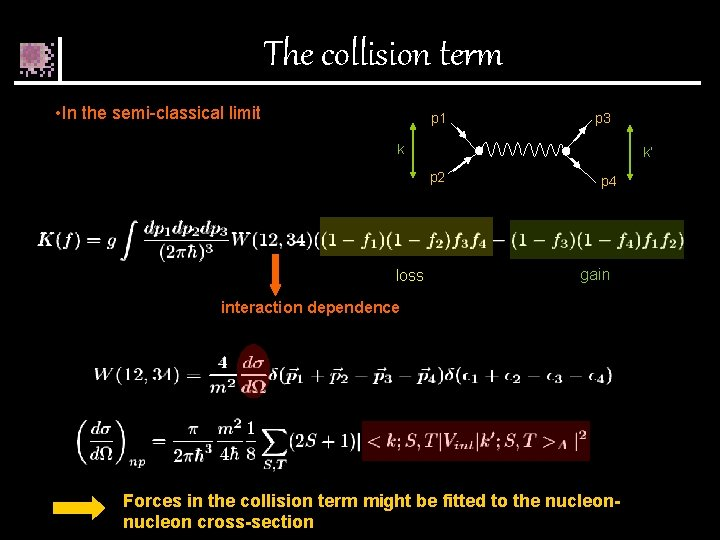The collision term • In the semi-classical limit p 1 p 3 k k’ p 2 loss p 4 gain interaction dependence Forces in the collision term might be fitted to the nucleon cross-section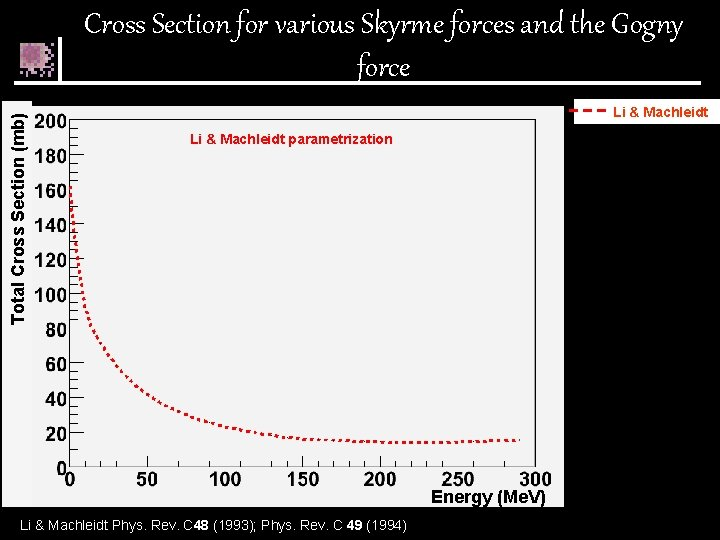Total Cross Section (mb) Cross Section for various Skyrme forces and the Gogny force Li & Machleidt parametrization Energy (Me. V) Li & Machleidt Phys. Rev. C 48 (1993); Phys. Rev. C 49 (1994)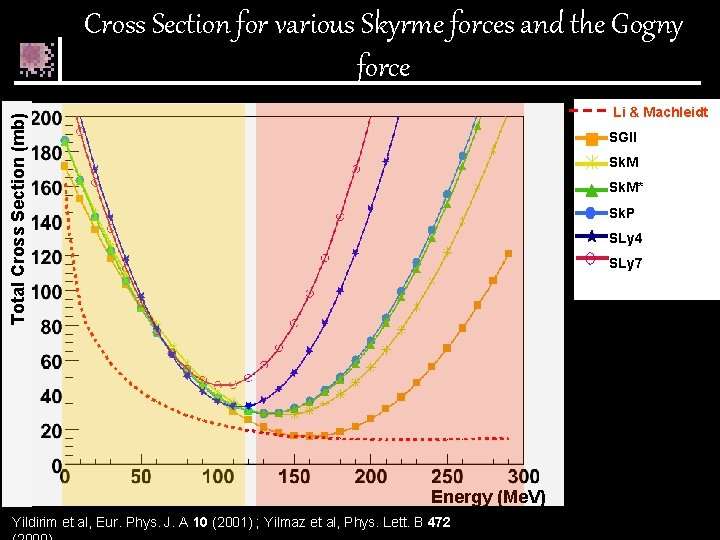Cross Section for various Skyrme forces and the Gogny force Total Cross Section (mb) Li & Machleidt SGII Sk. M* Sk. P SLy 4 SLy 7 Energy (Me. V) Yildirim et al, Eur. Phys. J. A 10 (2001) ; Yilmaz et al, Phys. Lett. B 472Cross Section for various Skyrme forces and the Gogny force Total Cross Section (mb) Li & Machleidt SGII Sk. M* Sk. P SLy 4 SLy 7 Gogny None of these forces reproduce nucleon-nucleon in medium crosssection new force energy (Me. V)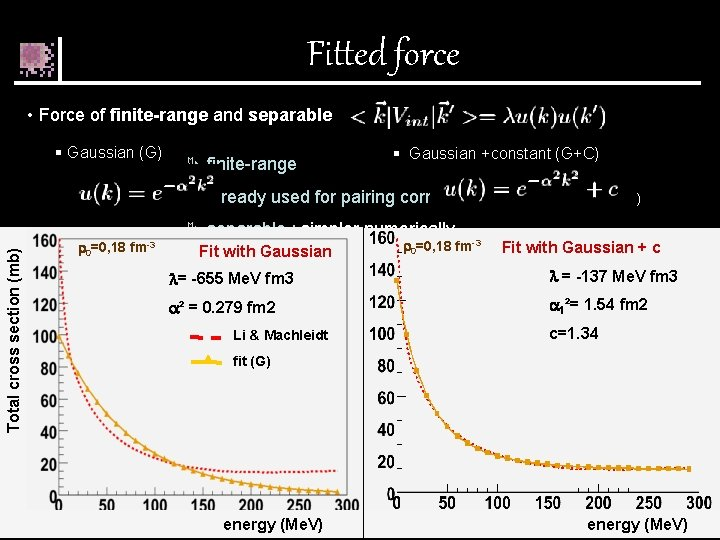Fitted force • Force of finite-range and separable § Gaussian (G) Ä finite-range § Gaussian +constant (G+C) Total cross section (mb) Ä already used for pairing correlations Duguet PRC 69 (2004) 0=0, 18 fm-3 Ä separable : simpler numerically Fit with Gaussian 0=0, 18 fm-3 Fit with Gaussian + c = -655 Me. V fm 3 = -137 Me. V fm 3 ² = 0. 279 fm 2 1²= 1. 54 fm 2 Li & Machleidt c=1. 34 fit (G) energy (Me. V)Density dependence Parameterization of Li & Machleidt E=50 Me. V Total cross section (mb) Li & Machleidt Phys. Rev. C 48 (1993); Phys. Rev. C 49 (1994) Li & Machleidt parameterization density (fm-3)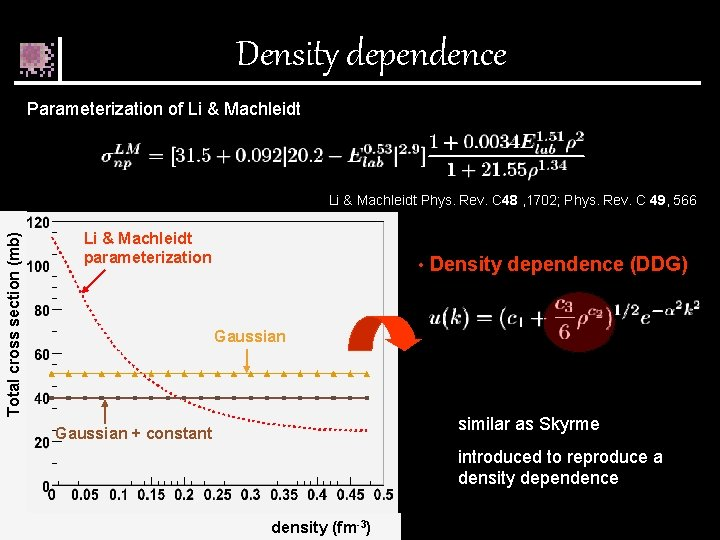Density dependence Parameterization of Li & Machleidt E=50 Me. V Total cross section (mb) Li & Machleidt Phys. Rev. C 48 , 1702; Phys. Rev. C 49, 566 Li & Machleidt parameterization • Density dependence (DDG) Gaussian similar as Skyrme Gaussian + constant introduced to reproduce a density dependence density (fm-3)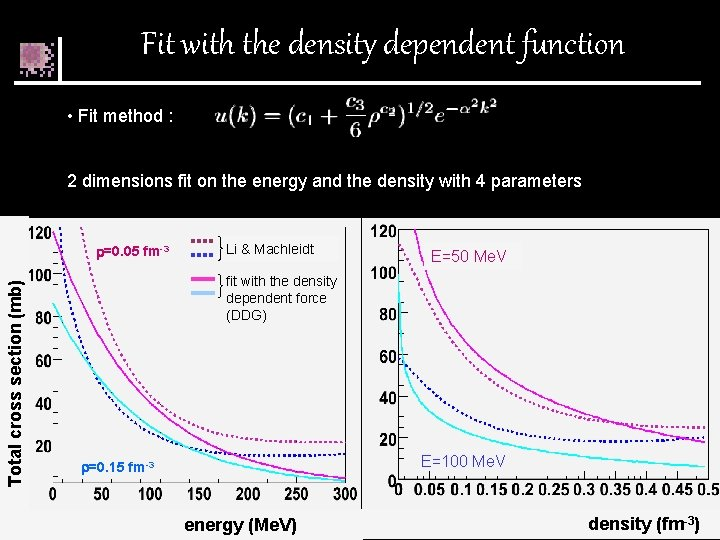Fit with the density dependent function • Fit method : 2 dimensions fit on the energy and the density with 4 parameters Total cross section (mb) =0. 05 fm-3 Li & Machleidt E=50 Me. V fit with the density dependent force (DDG) E=100 Me. V =0. 15 fm-3 energy (Me. V) density (fm-3)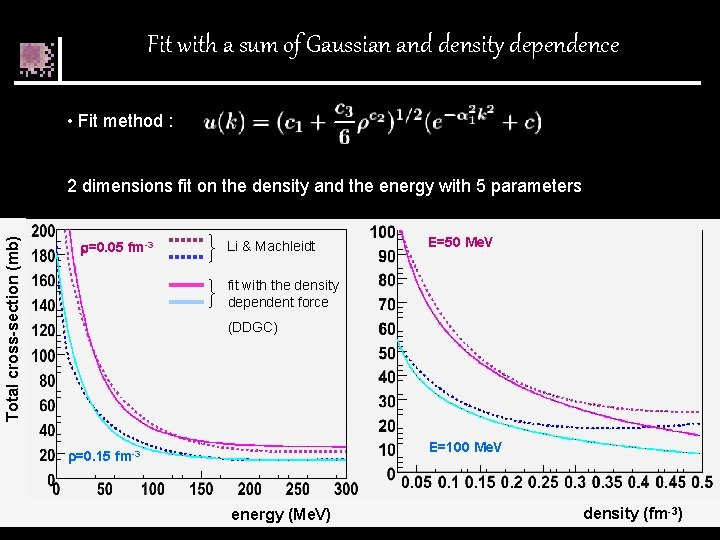Fit with a sum of Gaussian and density dependence • Fit method : Total cross-section (mb) 2 dimensions fit on the density and the energy with 5 parameters =0. 05 fm-3 Li & Machleidt E=50 Me. V fit with the density dependent force (DDGC) E=100 Me. V =0. 15 fm-3 energy (Me. V) density (fm-3)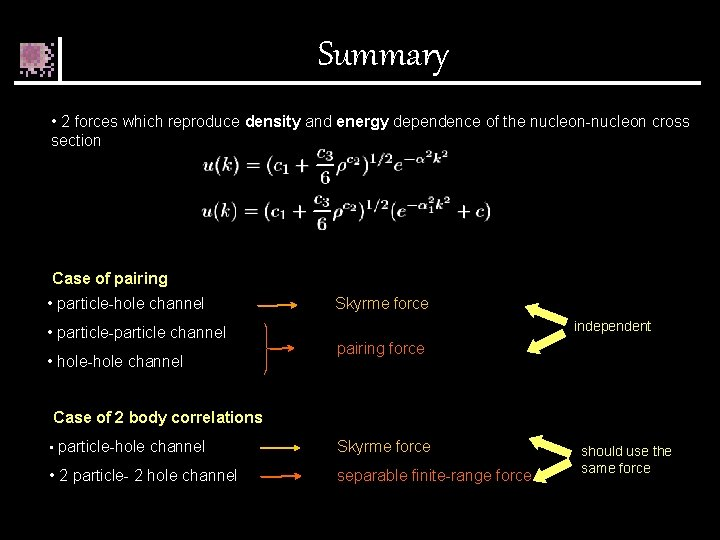Summary • 2 forces which reproduce density and energy dependence of the nucleon-nucleon cross section Case of pairing • particle-hole channel • particle-particle channel • hole-hole channel Skyrme force independent pairing force Case of 2 body correlations • particle-hole channel Skyrme force • 2 particle- 2 hole channel separable finite-range force should use the same forceComparison with the Skyrme force when k 0 and =0 • Skyrme Force t 0 • Gaussian t 1 -2 ² • Gaussian density dependent t 0 t 1 -2 ² t 3 c 3 Skyrme forces Gaussian (G) Gaussian+ constant (GC) Gaussian density dependent Gaussian+ constant density dependent Up to 10 parameters 2 parameters 3 parameters 4 parameters 5 parameters t 0=-2931 to -1057 Me. V. fm 3 - 655 Me. V. fm 3 - 748 Me. V. fm 3 - 2416 Me. V. fm 3 -2572 Me. V. fm 3 t 1=235 to 970 Me. V. fm 5 183 Me. V. fm 5 491 Me. V. fm 5 1038 Me. V. fm 5 1896 Me. V. fm 5 t 2=-556 to 107 Me. V. fm 5 Neglected t 3=8000 to 18708 Me. V. fm(3+3 ) 13107 Me. V. fm 3+3 13891 Me. V. fm 3+3 =1/6 to 1 0. 17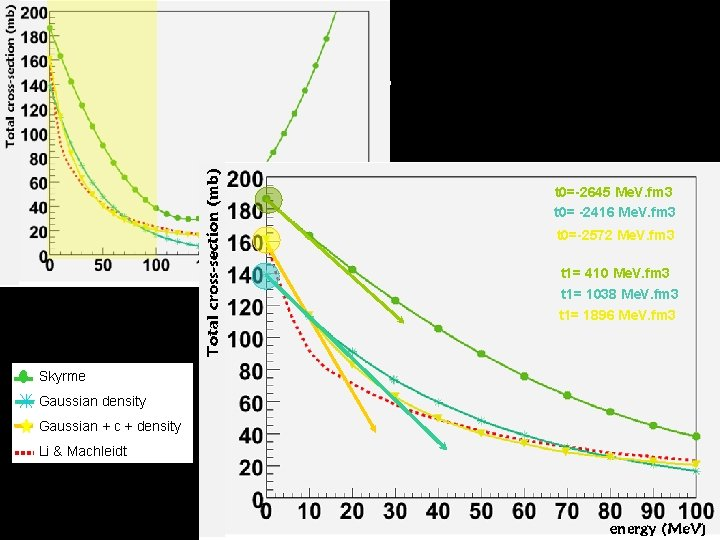t 0=-2645 Me. V. fm 3 t 0= -2416 Me. V. fm 3 t 0=-2572 Me. V. fm 3 t 1= 410 Me. V. fm 3 t 1= 1038 Me. V. fm 3 t 1= 1896 Me. V. fm 3 Skyrme Gaussian density Gaussian + c + density Li & MachleidtPerspectives • Time Dependent Density Matrix (TDDM) W. Cassing, S. J. Wang, Z. Phys. A, 328 (1987) § truncation of the BBGKY hierarchy § takes into account all the two body correlations (extension of HF) Skyrme force separable finite- range force • Perspectives : study of correlations between two neutrons in borromean nuclei Experiment planned for July 2006 at GANIL